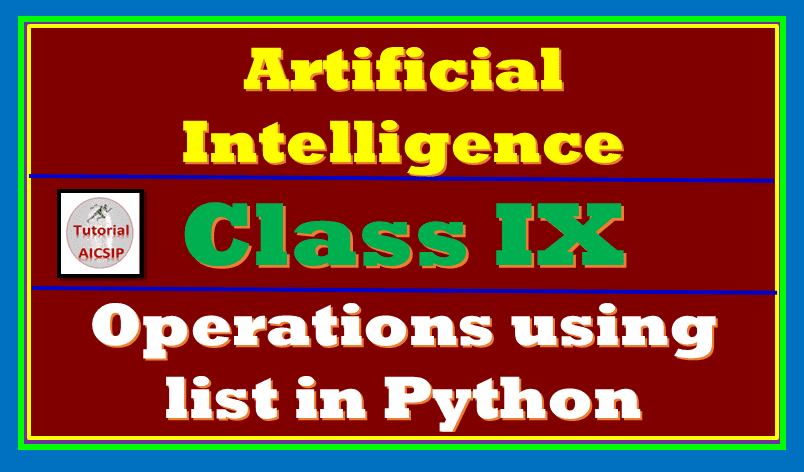In this article, you will get comprehensive notes on operations using list in python class 9. Now you are familiar with python programming. Sometimes we need to access more than one item in a single unit. For example, marks of the group of students, books of a class, etc. Python provides a list to perform such tasks. So let’s being now!

Topics Covered

## Introduction to list in python

A list of a sequence of similar or different values in a single unit of a different type. Observe the following line of code for a simple list in python:

`l=[10,30,50,70,90]`

A python list has the following common properties:

1. Each value of a list item is known as element or item.
2. Each value is separated by commas.
3. Values must be enlocsed by square brackets.
4. It is most versatile data type used in python.

## Operations using list in python class 9 for Artificial Intelligence

Now let’s start with how to perform Operations using the list in python class 9. The following operations can be performed on python lists: